Test: Polynomials- Case Based Type Questions

# Test: Polynomials- Case Based Type Questions - Class 8

Test Description

## 10 Questions MCQ Test Advance Learner Course: Mathematics (Maths) Class 8 - Test: Polynomials- Case Based Type Questions

Test: Polynomials- Case Based Type Questions for Class 8 2023 is part of Advance Learner Course: Mathematics (Maths) Class 8 preparation. The Test: Polynomials- Case Based Type Questions questions and answers have been prepared according to the Class 8 exam syllabus.The Test: Polynomials- Case Based Type Questions MCQs are made for Class 8 2023 Exam. Find important definitions, questions, notes, meanings, examples, exercises, MCQs and online tests for Test: Polynomials- Case Based Type Questions below.
Solutions of Test: Polynomials- Case Based Type Questions questions in English are available as part of our Advance Learner Course: Mathematics (Maths) Class 8 for Class 8 & Test: Polynomials- Case Based Type Questions solutions in Hindi for Advance Learner Course: Mathematics (Maths) Class 8 course. Download more important topics, notes, lectures and mock test series for Class 8 Exam by signing up for free. Attempt Test: Polynomials- Case Based Type Questions | 10 questions in 20 minutes | Mock test for Class 8 preparation | Free important questions MCQ to study Advance Learner Course: Mathematics (Maths) Class 8 for Class 8 Exam | Download free PDF with solutions
 1 Crore+ students have signed up on EduRev. Have you?
Test: Polynomials- Case Based Type Questions - Question 1

### Beti Bachao, Beti Padhao (BBBP) is a personal campaign of the Government of India that aims to generate awareness and improve the efficiency of welfare services intended for girls.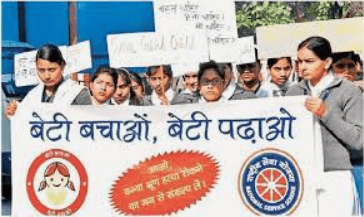In a school, a group of (x + y) teachers, (x2 + y2) girls and (x3 + y3) boys organised a campaign on Beti Bachao, Beti Padhao.Q. If in the group, there are 10 teachers and 58 girls, then what is the number of boys?

Detailed Solution for Test: Polynomials- Case Based Type Questions - Question 1

No. of teachers = x + y = 10

⇒ (x + y)2 = (10)2

⇒ x2 + y2 + 2xy = 100

[Since (a + b)2 = a2 + b2 + 2ab]

No. of students = (x2 + y2) = 58

⇒ 58 + 2xy = 100

⇒ 2xy = 100 – 58

⇒ 2xy = 42

⇒ xy = 42/2

⇒ xy = 21

Now, since (x + y)3 = [x3 + y3 + 3xy(x + y)]

⇒ (10)3 = [x3 + y3 + 3 × 21(10)]

⇒ 1000 = (x3 + y3 + 630)

⇒ 1000 – 630 = (x3 + y3)

⇒ (x3 + y3) = 370

Test: Polynomials- Case Based Type Questions - Question 2

### Beti Bachao, Beti Padhao (BBBP) is a personal campaign of the Government of India that aims to generate awareness and improve the efficiency of welfare services intended for girls.In a school, a group of (x + y) teachers, (x2 + y2) girls and (x3 + y3) boys organised a campaign on Beti Bachao, Beti Padhao.Q. Which is the correct identity?

Detailed Solution for Test: Polynomials- Case Based Type Questions - Question 2
Here, Correct identity is (a + b)2 = a2 + b2 + 2ab.
Test: Polynomials- Case Based Type Questions - Question 3

### Beti Bachao, Beti Padhao (BBBP) is a personal campaign of the Government of India that aims to generate awareness and improve the efficiency of welfare services intended for girls.In a school, a group of (x + y) teachers, (x2 + y2) girls and (x3 + y3) boys organised a campaign on Beti Bachao, Beti Padhao.Q. Which mathematical concept is used here?

Detailed Solution for Test: Polynomials- Case Based Type Questions - Question 3
Here, we used Polynomials Concept.

In mathematics, a polynomial is an expression consisting of indeterminates (also called variables) and coefficients, that involves only the operations of addition, subtraction, multiplication, and non-negative integer exponentiation of variables.

Test: Polynomials- Case Based Type Questions - Question 4

Beti Bachao, Beti Padhao (BBBP) is a personal campaign of the Government of India that aims to generate awareness and improve the efficiency of welfare services intended for girls.In a school, a group of (x + y) teachers, (x2 + y2) girls and (x3 + y3) boys organised a campaign on Beti Bachao, Beti Padhao.

Q. (x – y)3 =

Detailed Solution for Test: Polynomials- Case Based Type Questions - Question 4

We know that (x - y)3 can be written as

(x - y)(x - y)(x - y)

We know that (x - y)(x - y) can be multiplied and written as

= x2 - xy - yx + y2 (x - y)

= x2 - 2xy + y2 (x - y)

= x3 - 2x2y + xy2 - yx2 + 2xy2 - y3

= x3 – y3 – 2xy(x – y)

Test: Polynomials- Case Based Type Questions - Question 5

Beti Bachao, Beti Padhao (BBBP) is a personal campaign of the Government of India that aims to generate awareness and improve the efficiency of welfare services intended for girls.In a school, a group of (x + y) teachers, (x2 + y2) girls and (x3 + y3) boys organised a campaign on Beti Bachao, Beti Padhao.

Q. Using part (D), find (x2 – y2) if x – y = 23.

Detailed Solution for Test: Polynomials- Case Based Type Questions - Question 5
Given x – y = 23

Also, x + y = 10

x2 – y2 = (x + y)(x – y)

= 10 × 23

= 230

Test: Polynomials- Case Based Type Questions - Question 6

National Association For The Blind (NAB) aimed to empower and well-inform visually challenged population of our country, thus enabling them to lead a life of dignity and productivity.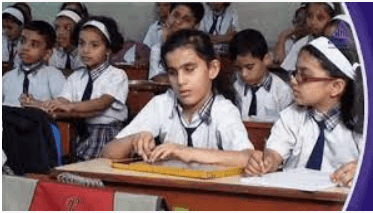Ravi donated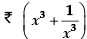to NAB. When his cousin asks to tell the amount donated by him, he just gave the below hint.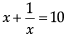Q. Find the amount donated by Ravi.

Detailed Solution for Test: Polynomials- Case Based Type Questions - Question 6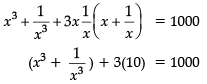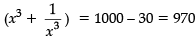Test: Polynomials- Case Based Type Questions - Question 7

National Association For The Blind (NAB) aimed to empower and well-inform visually challenged population of our country, thus enabling them to lead a life of dignity and productivity.Ravi donatedto NAB. When his cousin asks to tell the amount donated by him, he just gave the below hint.

Q. (x – y)3 =

Detailed Solution for Test: Polynomials- Case Based Type Questions - Question 7
We know that (x - y)3 can be written as

(x - y)(x - y)(x - y)

We know that (x - y)(x - y) can be multiplied and written as

x2 - xy - yx + y2 (x - y)

= x2 - 2xy + y2 (x - y)

= x3 - 2x2y + xy2 - yx2 + 2xy2 - y3

= x3 – y3 – 3xy(x – y)

Test: Polynomials- Case Based Type Questions - Question 8

National Association For The Blind (NAB) aimed to empower and well-inform visually challenged population of our country, thus enabling them to lead a life of dignity and productivity.Ravi donatedto NAB. When his cousin asks to tell the amount donated by him, he just gave the below hint.

Q. (x + a)(x + b) = x2 + ................ x + ab

Detailed Solution for Test: Polynomials- Case Based Type Questions - Question 8
(x + a)(x + b) = x2 + (a + b)x + ab
Test: Polynomials- Case Based Type Questions - Question 9

National Association For The Blind (NAB) aimed to empower and well-inform visually challenged population of our country, thus enabling them to lead a life of dignity and productivity.Ravi donatedto NAB. When his cousin asks to tell the amount donated by him, he just gave the below hint.

Q. Which mathematical concept is involved in the above situation?

Detailed Solution for Test: Polynomials- Case Based Type Questions - Question 9
Here, we used Polynomials Concept.

In mathematics, a polynomial is an expression consisting of indeterminates (also called variables) and coefficients, that involves only the operations of addition, subtraction, multiplication, and non-negative integer exponentiation of variables.

Test: Polynomials- Case Based Type Questions - Question 10

National Association For The Blind (NAB) aimed to empower and well-inform visually challenged population of our country, thus enabling them to lead a life of dignity and productivity.Ravi donatedto NAB. When his cousin asks to tell the amount donated by him, he just gave the below hint.

Q. Find the amount donated by Ravi if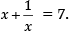Detailed Solution for Test: Polynomials- Case Based Type Questions - Question 10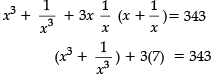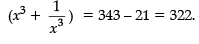## Advance Learner Course: Mathematics (Maths) Class 8

13 videos|62 docs|24 tests
Information about Test: Polynomials- Case Based Type Questions Page
In this test you can find the Exam questions for Test: Polynomials- Case Based Type Questions solved & explained in the simplest way possible. Besides giving Questions and answers for Test: Polynomials- Case Based Type Questions, EduRev gives you an ample number of Online tests for practice

## Advance Learner Course: Mathematics (Maths) Class 8

13 videos|62 docs|24 tests